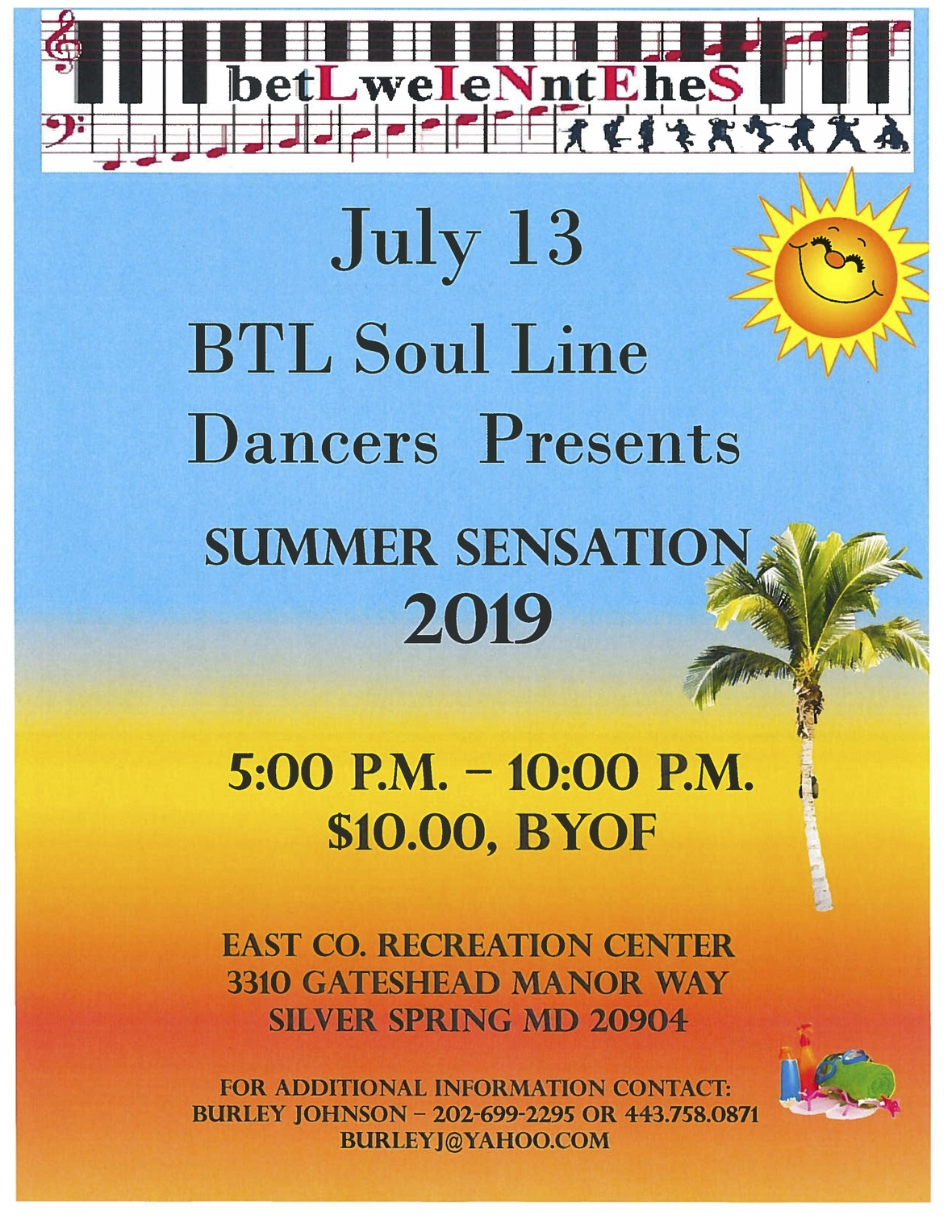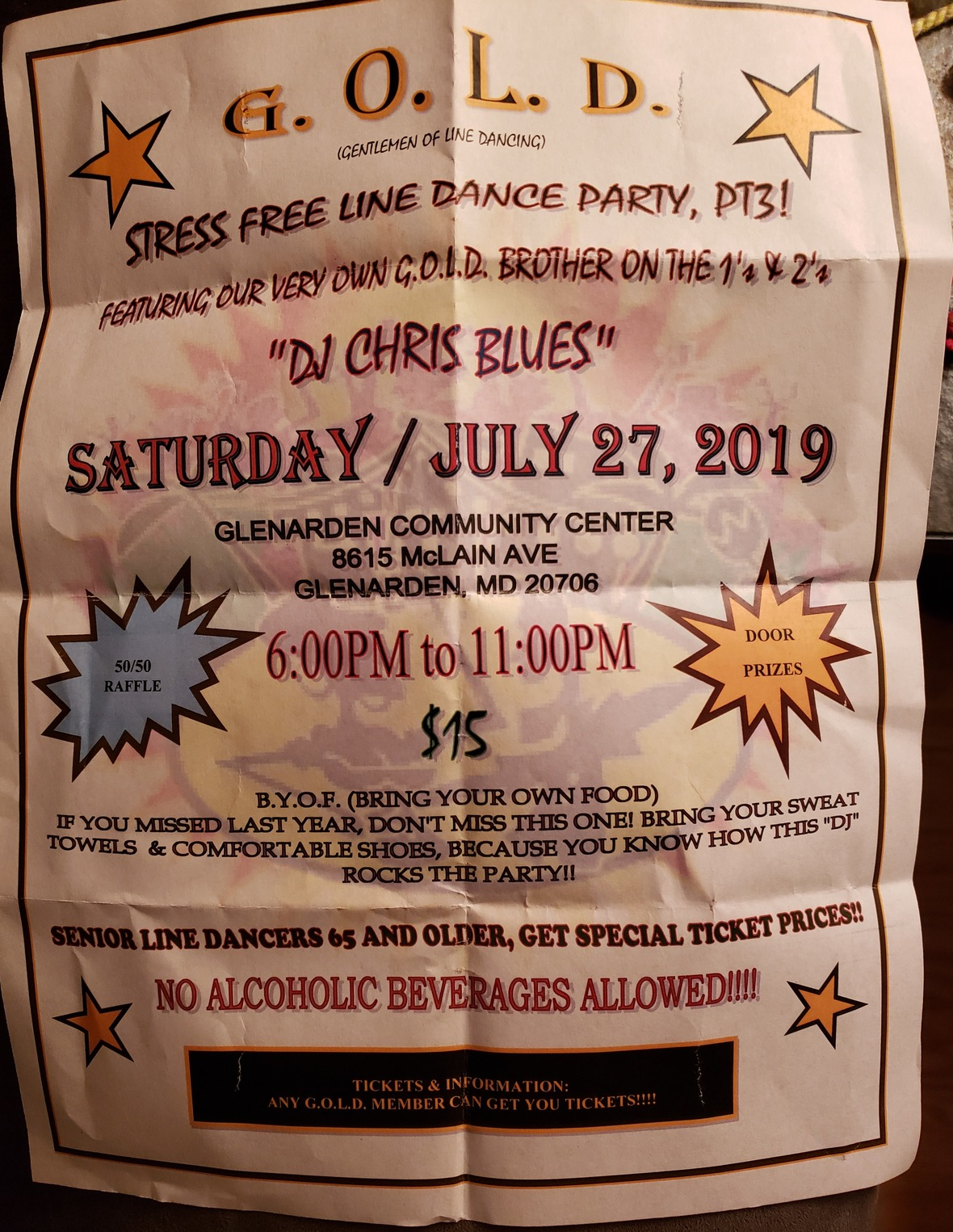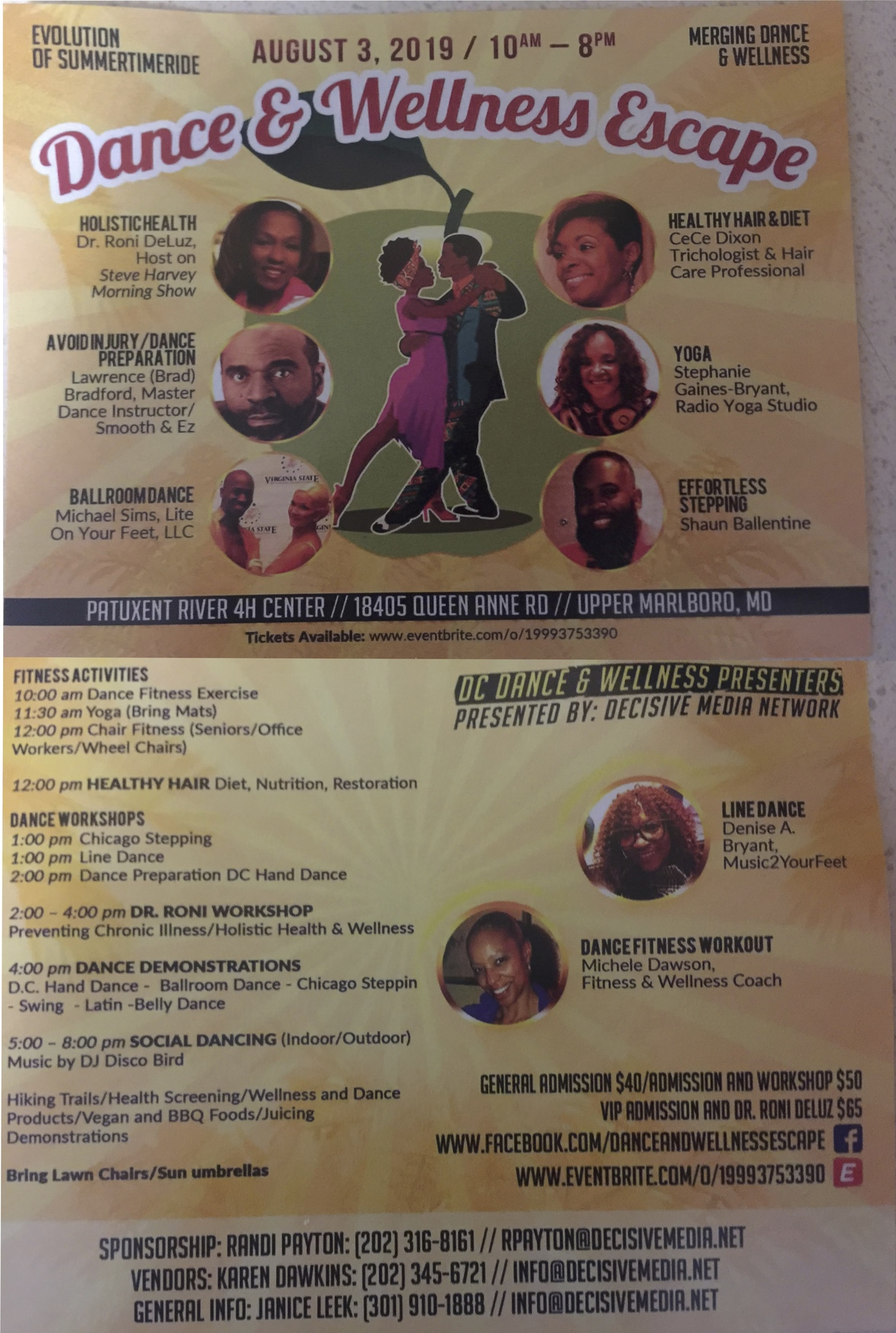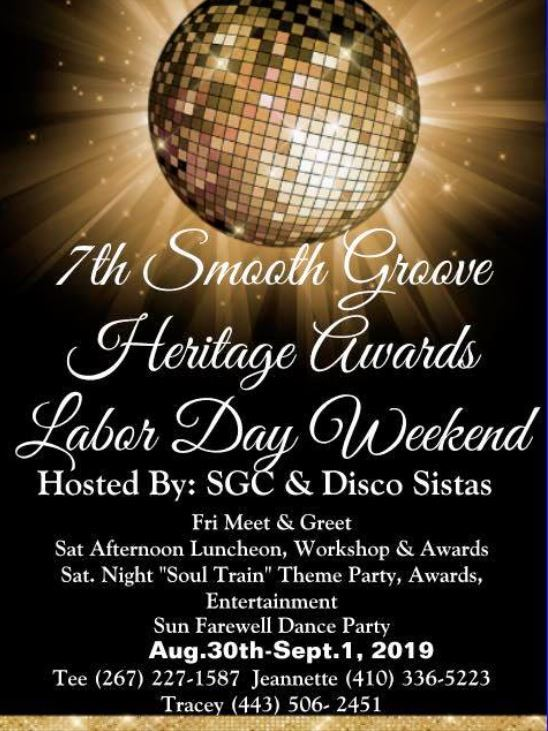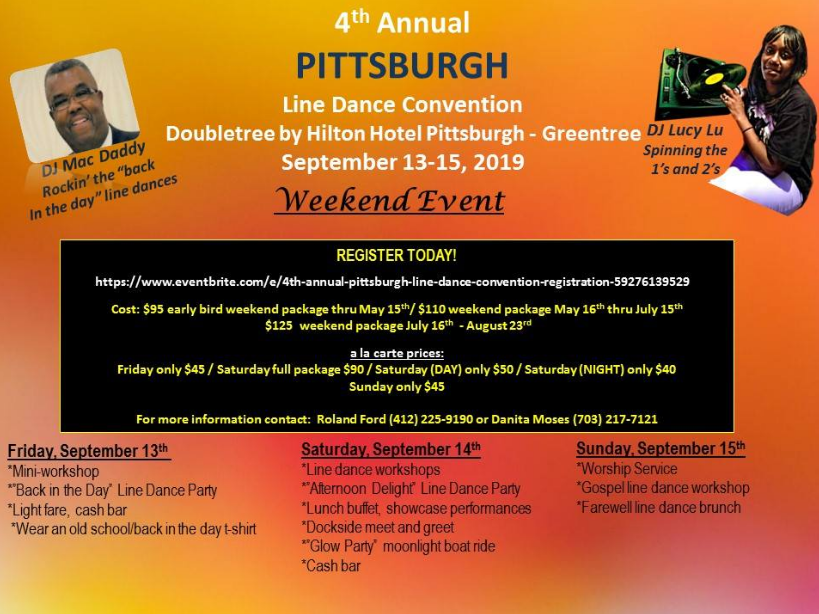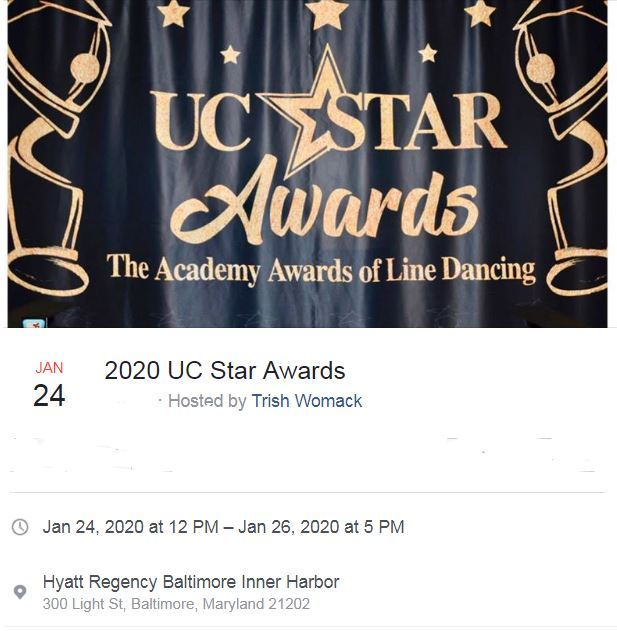## Health Tips for the 4th!

 /* styles */ No Matter The Day - It Will Be Music2YourFeet! -Denise Bryant, Founder
 table div table+table+table+table+table div table{width:100%;padding:0}table div table+table+table+table+table div table img{width:96.23%;padding:0;float:none}table div table+table+table+table+table div table td{width:100%;padding:0 1.88% 18px}/* styles *//* styles */

## A few health tips for July 4th

 table div table+table+table+table+table+table+table+table+table+table div table{width:100%;padding:0}table div table+table+table+table+table+table+table+table+table+table div table img{width:96.23%;padding:0;float:none}table div table+table+table+table+table+table+table+table+table+table div table td{width:100%;padding:0 1.88% 18px}/* styles */table div table+table+table+table+table+table+table+table+table+table+table div table{width:100%;padding:0}table div table+table+table+table+table+table+table+table+table+table+table div table img{width:96.23%;padding:0;float:none}table div table+table+table+table+table+table+table+table+table+table+table div table td{width:100%;padding:0 1.88% 18px}/* styles */## Denise's Corner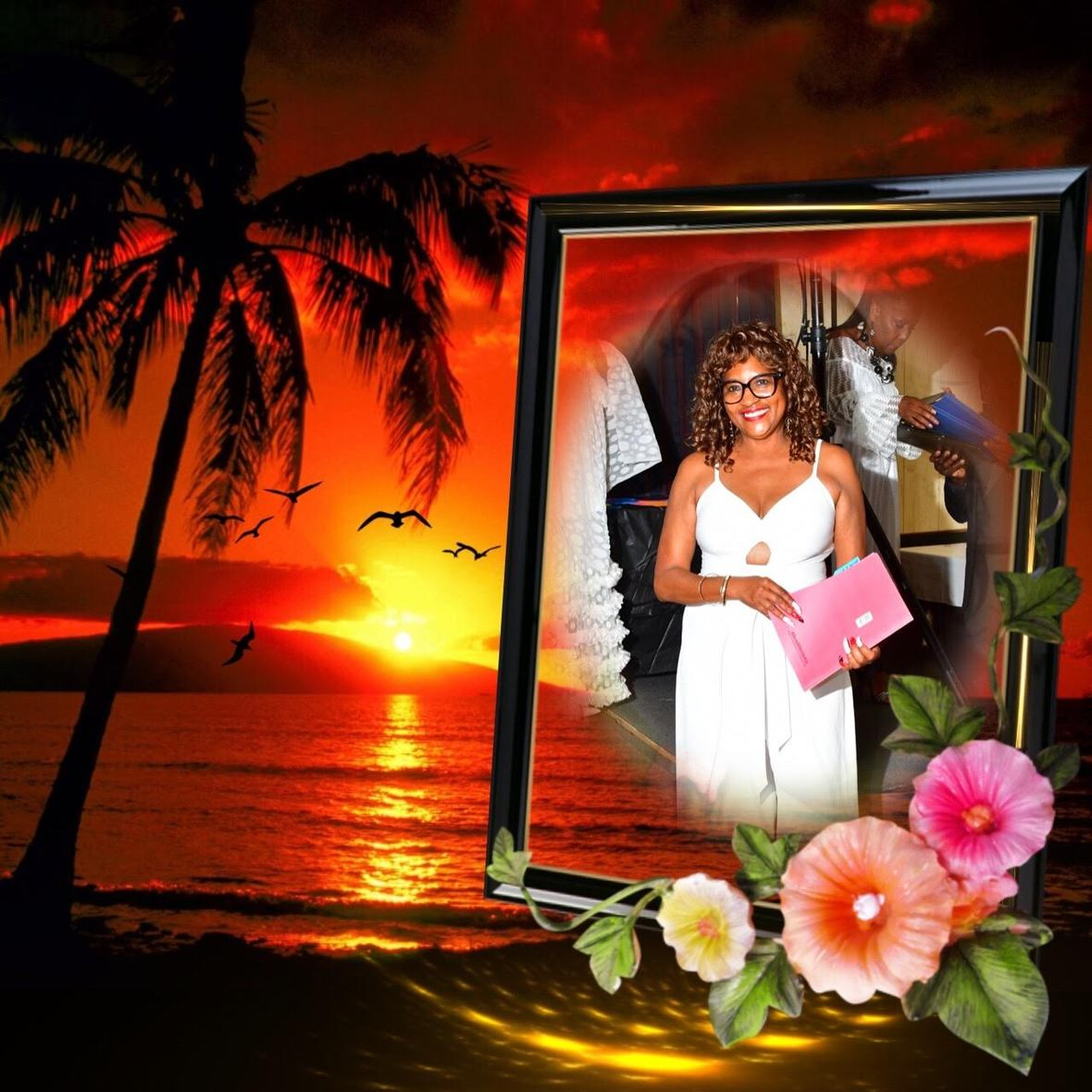Friday, September 13-15th, Pittsburgh Line Dance Event. No classes at Cedar Hgts CC, and Goodluck CC.

In the Spotlight
We would love to spotlight your event or an individual in the community.

We will feature someone or an event(s) in the next edition of Denise's corner.

Donations not required but appreciated.

 table div table+table+table+table+table+table+table+table+table+table+table+table+table+table+table div table{width:100%;padding:0}table div table+table+table+table+table+table+table+table+table+table+table+table+table+table+table div table img{width:96.23%;padding:0;float:none}table div table+table+table+table+table+table+table+table+table+table+table+table+table+table+table div table td{width:100%;padding:0 1.88% 18px}/* styles */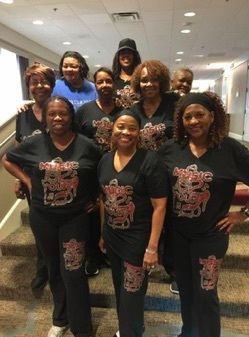Music2YourFeet Workout Suit (2 Piece)

\$40 for set, separate \$20 each,
come in black and gray only, stretch material...size from small to 2x pants and 3X shirt, may use Cash App \$seafo1 to pay, or cash, sorry no paypal and no checks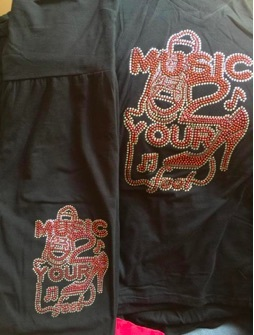Music2YourFeetWorkout Suit (pant and t-shirt)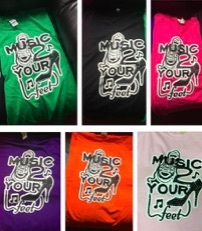Music2YourFeet T-Shirt

\$15 each, may use Cash App \$seafo1 to pay, or cash, sorry no paypal and no checks

 table div table+table+table+table+table+table+table+table+table+table+table+table+table+table+table+table+table+table+table+table div table{width:100%;padding:0}table div table+table+table+table+table+table+table+table+table+table+table+table+table+table+table+table+table+table+table+table div table img{width:96.23%;padding:0;float:none}table div table+table+table+table+table+table+table+table+table+table+table+table+table+table+table+table+table+table+table+table div table td{width:100%;padding:0 1.88% 18px}/* styles */## Happy Birthday July Babies!

 /* styles */ Happy Birthday to all the July Babies, enjoy your Special Day!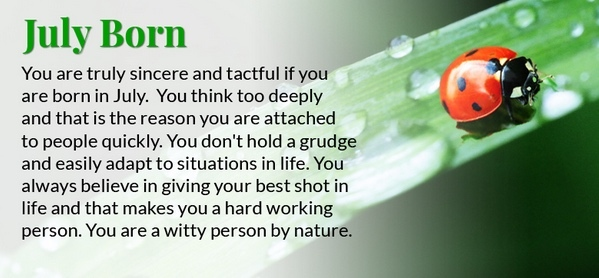table div table+table+table+table+table+table+table+table+table+table+table+table+table+table+table+table+table+table+table+table+table+table+table+table+table div table{width:100%;padding:0}table div table+table+table+table+table+table+table+table+table+table+table+table+table+table+table+table+table+table+table+table+table+table+table+table+table div table img{width:96.23%;padding:0;float:none}table div table+table+table+table+table+table+table+table+table+table+table+table+table+table+table+table+table+table+table+table+table+table+table+table+table div table td{width:100%;padding:0 1.88% 18px}/* styles */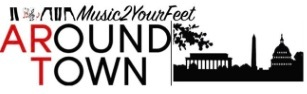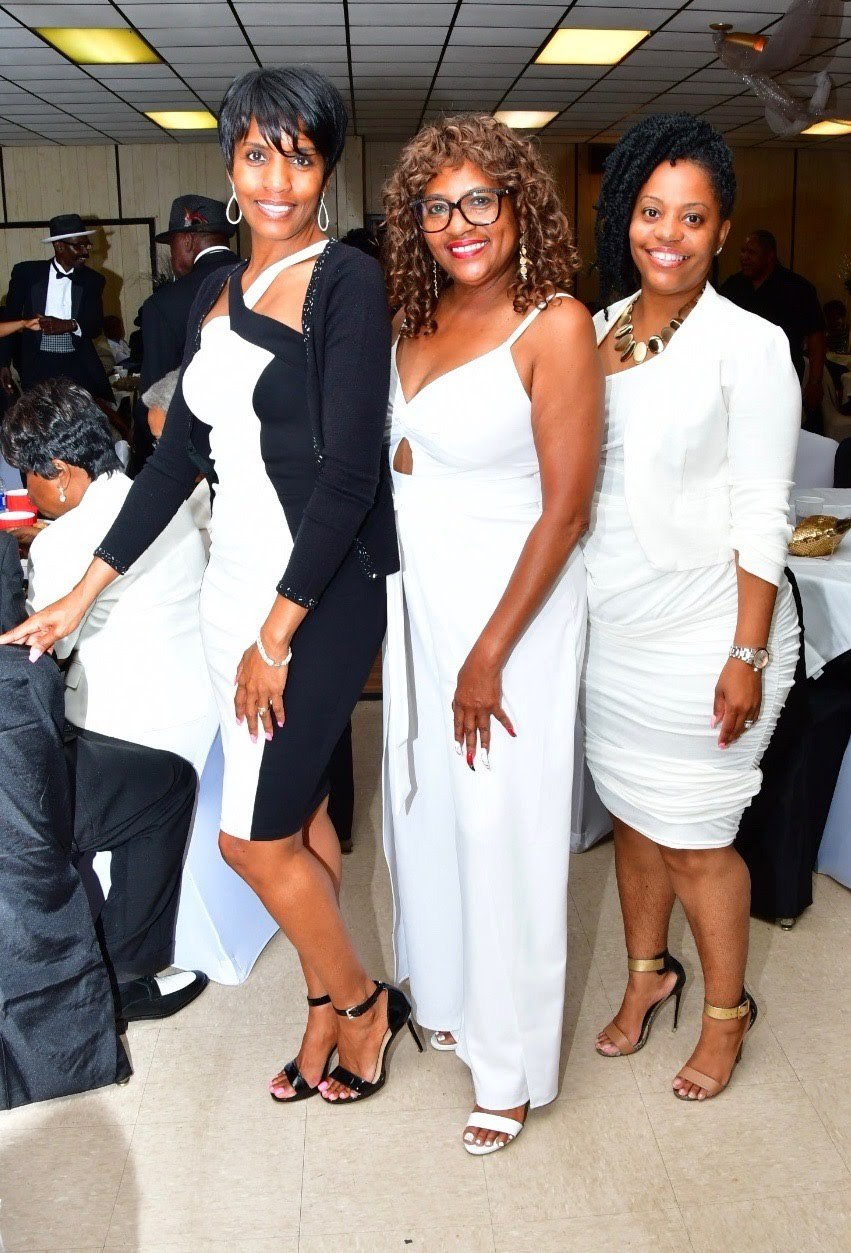Music2YourFeet visits many areas within the community, centers, parks and other events - spreading our love of line dancing. We capture every moment with video and photos. Please check out our photo gallery each month - you may find yourself in them - "Aroundtown"!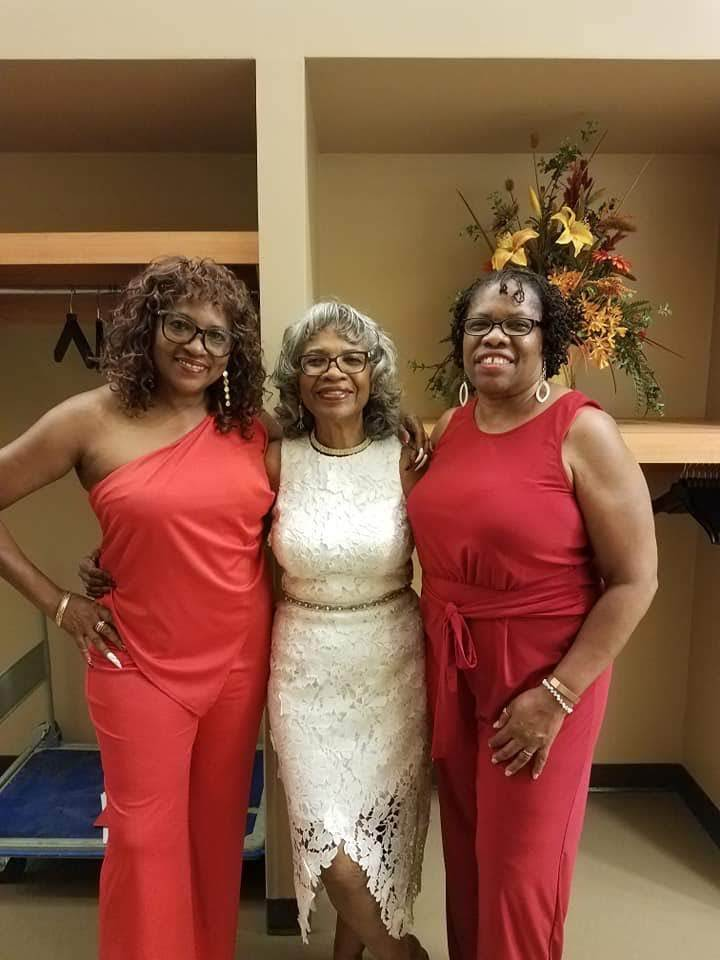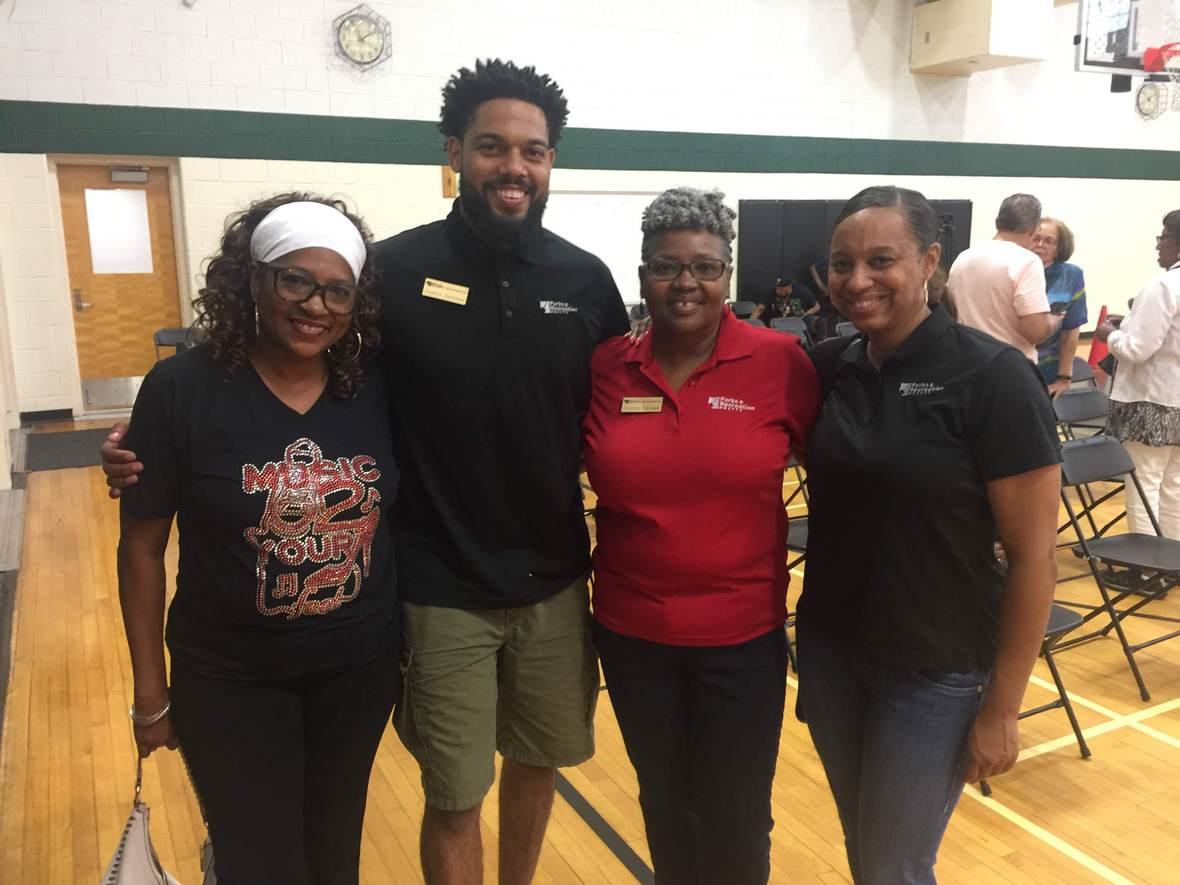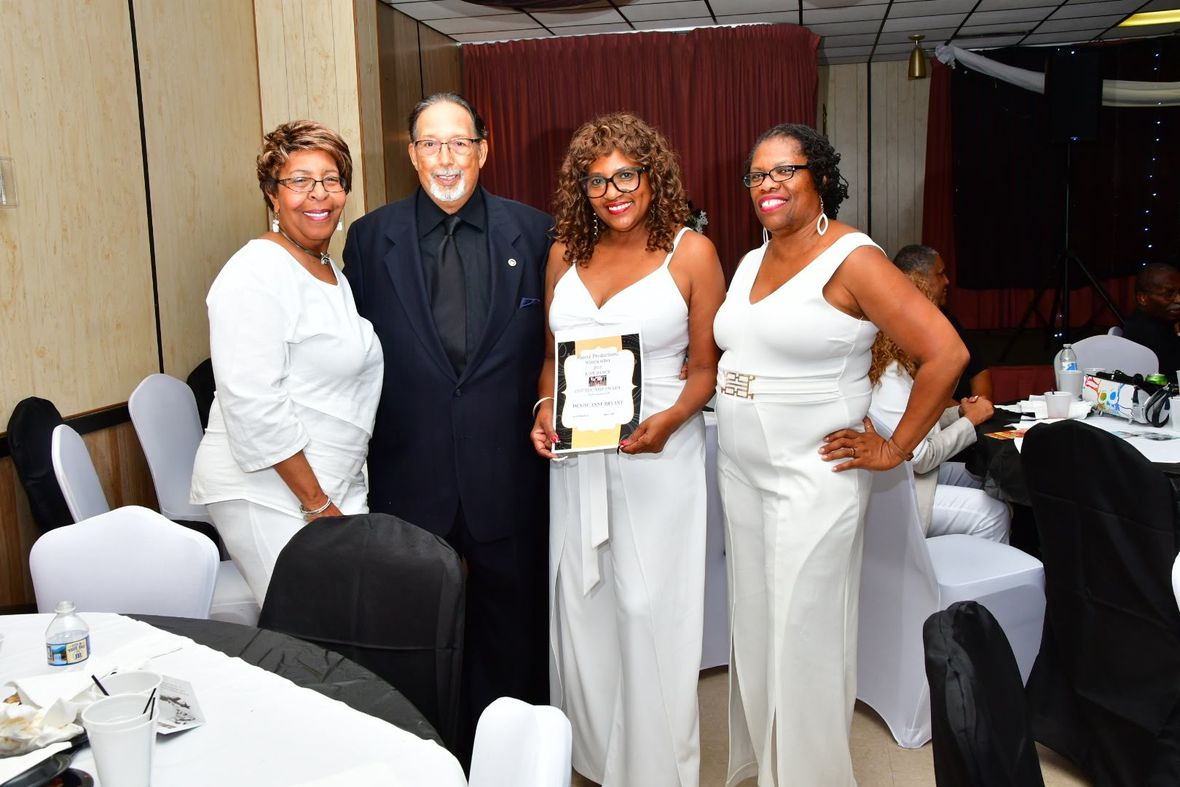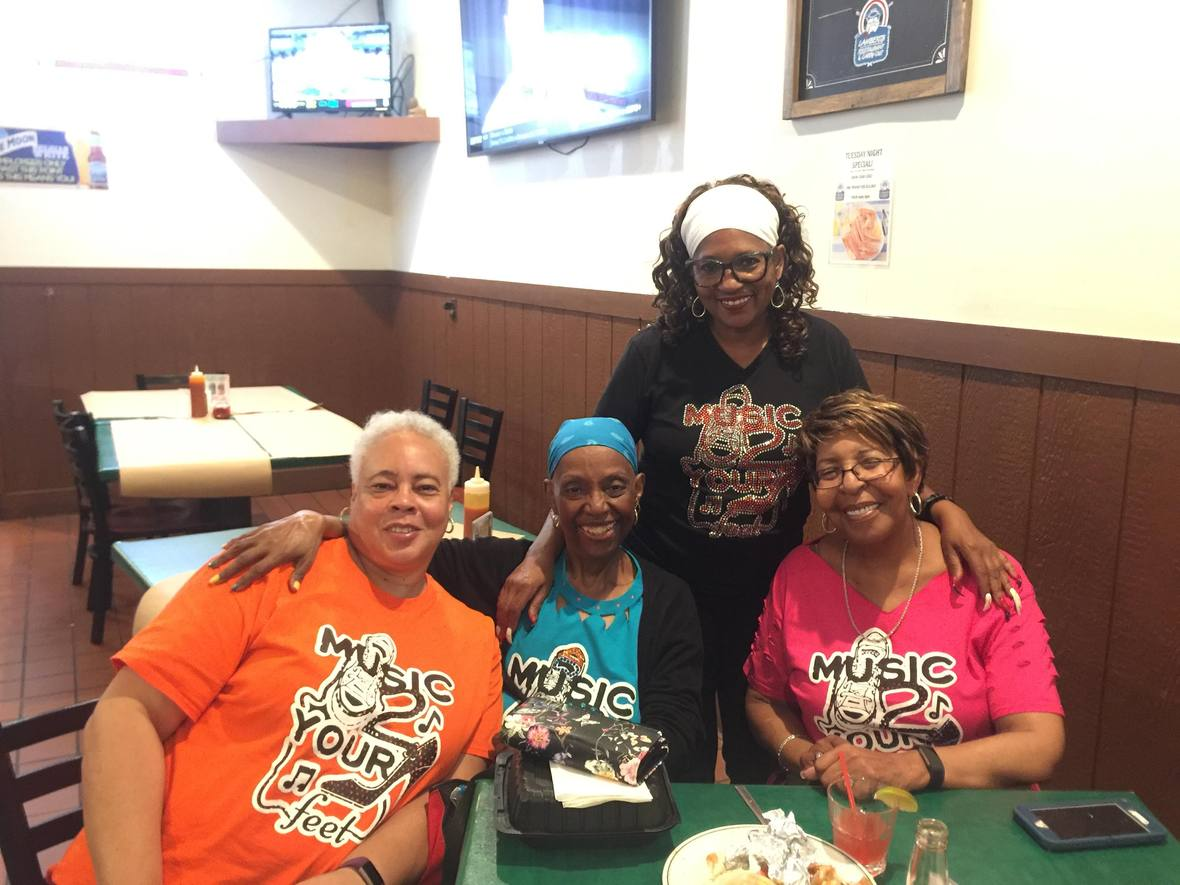table div table+table+table+table+table+table+table+table+table+table+table+table+table+table+table+table+table+table+table+table+table+table+table+table+table+table+table+table+table+table+table div table{width:100%;padding:0}table div table+table+table+table+table+table+table+table+table+table+table+table+table+table+table+table+table+table+table+table+table+table+table+table+table+table+table+table+table+table+table div table img{width:96.23%;padding:0;float:none}table div table+table+table+table+table+table+table+table+table+table+table+table+table+table+table+table+table+table+table+table+table+table+table+table+table+table+table+table+table+table+table div table td{width:100%;padding:0 1.88% 18px}/* styles */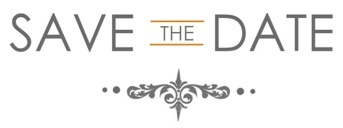/* styles */ DAB Travel Party Bus Trip - _*This Trip is SOLD OUT* August 23-25, 2019 Virginia Beach M2YF Holiday Party December 21, 2019
 table div table+table+table+table+table+table+table+table+table+table+table+table+table+table+table+table+table+table+table+table+table+table+table+table+table+table+table+table+table+table+table+table+table+table div table{width:100%;padding:0}table div table+table+table+table+table+table+table+table+table+table+table+table+table+table+table+table+table+table+table+table+table+table+table+table+table+table+table+table+table+table+table+table+table+table div table img{width:96.23%;padding:0;float:none}table div table+table+table+table+table+table+table+table+table+table+table+table+table+table+table+table+table+table+table+table+table+table+table+table+table+table+table+table+table+table+table+table+table+table div table td{width:100%;padding:0 1.88% 18px}/* styles */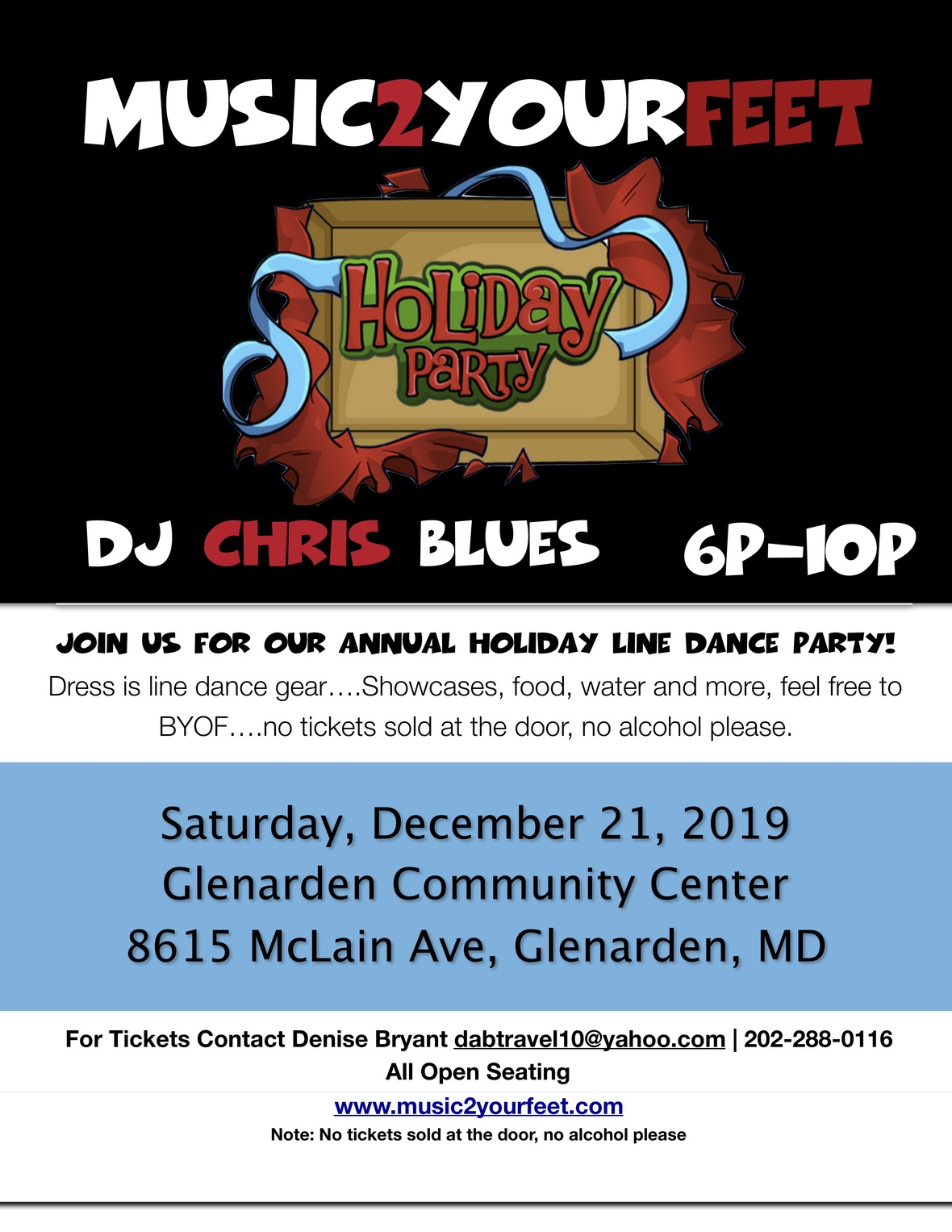table div table+table+table+table+table+table+table+table+table+table+table+table+table+table+table+table+table+table+table+table+table+table+table+table+table+table+table+table+table+table+table+table+table+table+table+table div table{width:100%;padding:0}table div table+table+table+table+table+table+table+table+table+table+table+table+table+table+table+table+table+table+table+table+table+table+table+table+table+table+table+table+table+table+table+table+table+table+table+table div table img{width:96.23%;padding:0;float:none}table div table+table+table+table+table+table+table+table+table+table+table+table+table+table+table+table+table+table+table+table+table+table+table+table+table+table+table+table+table+table+table+table+table+table+table+table div table td{width:100%;padding:0 1.88% 18px}/* styles */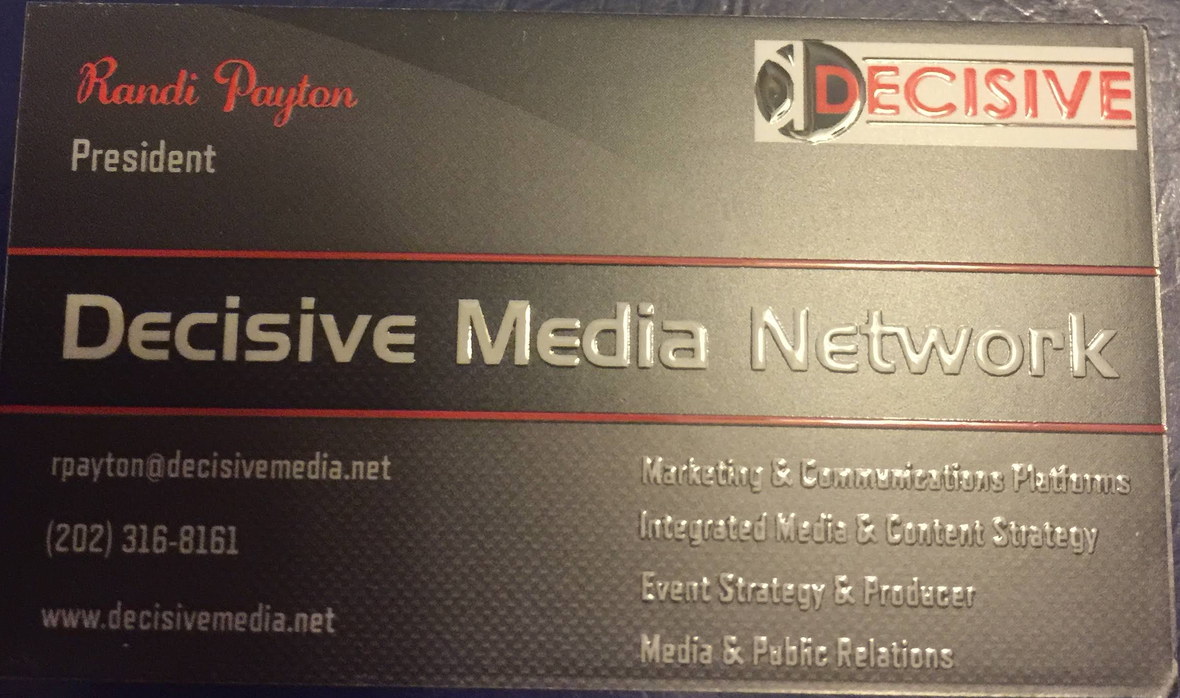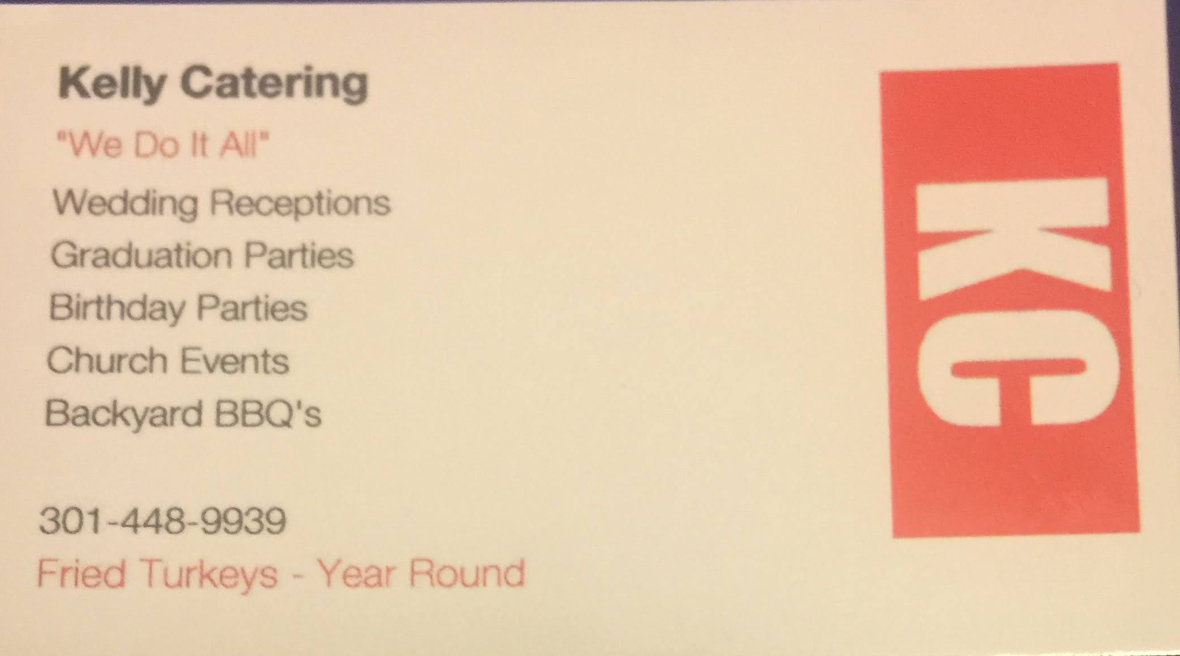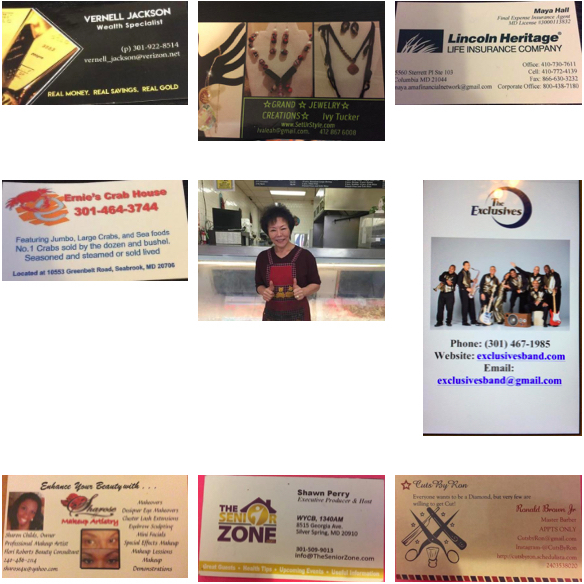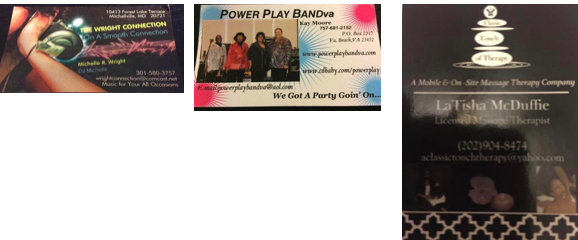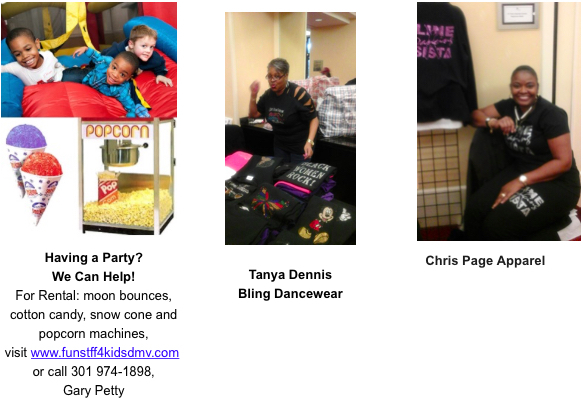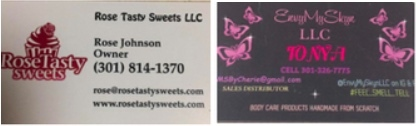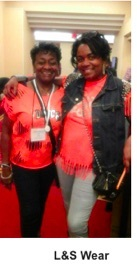# Thank you for your continued support!

 table div table+table+table+table+table+table+table+table+table+table+table+table+table+table+table+table+table+table+table+table+table+table+table+table+table+table+table+table+table+table+table+table+table+table+table+table+table+table+table+table+table+table+table+table+table+table+table div table{width:100%;padding:0}table div table+table+table+table+table+table+table+table+table+table+table+table+table+table+table+table+table+table+table+table+table+table+table+table+table+table+table+table+table+table+table+table+table+table+table+table+table+table+table+table+table+table+table+table+table+table+table div table img{width:96.23%;padding:0;float:none}table div table+table+table+table+table+table+table+table+table+table+table+table+table+table+table+table+table+table+table+table+table+table+table+table+table+table+table+table+table+table+table+table+table+table+table+table+table+table+table+table+table+table+table+table+table+table+table div table td{width:100%;padding:0 1.88% 18px}/* styles */## Music2YourFeet Class Schedule Closings (Spring-Summer)No line dance classes the month of August, for music2yourfeet

Lake Arbor Community Center, Last day of class, & Day Party, June 12th, Line dance party,10a-2p...No summer classes

Cedar Hgts Community Center Line dance Day Party, June 11th, 10a-1pm...open to all..Pat Duncan, Garland Christopher, & Denise Bryant will teach..Dj Garland

Cedar Hgts Community Center last day for Friday class is June 28th, last day for Tuesday class is July 30th.

Glendale Community Center last day at Glendale, June 4th, start Palmer Park Community Center, June 18th 11-12:30, for the month of June & July only.

Good Luck Community Center Line dance Day Party Friday, June 14th, 11:00-2:00pm- open to all. Will Baker, Dj, & Instructors, Laura Brown, Garland Christopher

Good Luck Community Center, Wednesday Evening class end on June 19th..No summer classes

Good Luck Community Center, Friday Senior class last day is June 21st...No summer classes

## Music2YourFeet Classes

 /* styles */ Monday Lake Arbor Community Center 10100 Lake Arbor Way, Mitchellville, Md. 20721 10:00am-11:30am Calling all Beginners & Seniors, come out for some fun and exercise with Denise Tuesday Cedar Heights Community Center 1200 Glen Willow Drive Seat Pleasant, MD 9:00am-10:30am \$6, Intermediate Tuesday Glenn Dale Community Center 11901 Glenn Dale Blvd, Glenn Dale, MD 20769 11-12:30pm Beginner \$20 for residents and \$24 for Non-residents. \$5 drop in fee. Beginner Wednesday Good Luck Community Ctr. 6:30-8:00pm 8601 Good Luck Rd, Lanham, Md. 20706 Beginner Friday Ceder Heights Community Center 9am-10:30am 1200 Glen Willow Dr, Capitol Heights, MD 20743 Beginner Friday Senior Day Good Luck Community Center (on-going) 8601 Good Luck Rd Lanham, Md. 11:30am-1:30pm Seniors 60+ FREE, under 60 yrs. Old \$6 Music2YourFeet Private Lessons Available
 table div table+table+table+table+table+table+table+table+table+table+table+table+table+table+table+table+table+table+table+table+table+table+table+table+table+table+table+table+table+table+table+table+table+table+table+table+table+table+table+table+table+table+table+table+table+table+table+table+table+table+table+table div table{width:100%;padding:0}table div table+table+table+table+table+table+table+table+table+table+table+table+table+table+table+table+table+table+table+table+table+table+table+table+table+table+table+table+table+table+table+table+table+table+table+table+table+table+table+table+table+table+table+table+table+table+table+table+table+table+table+table div table img{width:96.23%;padding:0;float:none}table div table+table+table+table+table+table+table+table+table+table+table+table+table+table+table+table+table+table+table+table+table+table+table+table+table+table+table+table+table+table+table+table+table+table+table+table+table+table+table+table+table+table+table+table+table+table+table+table+table+table+table+table div table td{width:100%;padding:0 1.88% 18px}/* styles */## DMV Monthly Dance Socials

 /* styles */ First Sunday of Each Month Sunday Line Dance Social at Glenarden Comm Ctr. 3pm - 7pm \$6 for Res & Non-Res. For more information, call 301-772-3151 First Friday Line Dance Socials with April Love Jones Southern Regional Technology and Recreation Complex, 6pm-9:30pm includes light refreshments, \$5 resident \$6 non resident First and Third Friday Delight featuring DJ Zone @ VFW Post 7916 (upstairs), 204 Mill Street Occoquan, Va 22125. Time: 7:30 PM to 11:30 PM. Cost: \$10. Open dancing, Cash Bar, Bring your own Food or purchase from the VFW. Third Friday S.O.S. (Seniors on Step) Old School/New School Line dance Social Friday November 16, 7-10:30pm..Fit Intensity 5641 General Washington Drive- Unit C, Alexandria, Va 22312 Admission \$5pp- food for sale NO Alcohol permitted First Saturday of Each Month - Toast it Up Productions - Shut Up & Dance, 1:00-5:00pm, Elk's Lodge, 7350 Temple Hills, Md. BYOF, \$7.00 Every Second Friday, \$5 6p-9p. Learn the latest line dances with Peytrienne! All are welcome, water provided, Soul Line & Hand dance Party, White Oak Community Center, 1700 April Lane Silver Spring, Md. 20904 Every Second Sunday Spectacular Sunday's with DJ Zone Elks Lodge of Camp Springs 7350 Temple Hills Rd Temple Hills Md, \$10 Hand Dance, Bop, Line Dance & more 6p-10p Every Tuesday of the Month (except during early voting and election weeks) DMV Tuesday Dance Vibe DJ Zone 1st and 3rd Tuesday DJ Moe 2nd and 4th Tuesday VFW Post 8950 8950 Goodluck Rd Lanham MD, \$6 Hand Dance, Bop, Line Dance & more 6p-10p Fourth Friday of the Month - Style & Rhythm, 4th Friday social ,\$5, Ridgley's Run Community Ctr. 8400 Mission Road, Jessup, Md.
 table div table+table+table+table+table+table+table+table+table+table+table+table+table+table+table+table+table+table+table+table+table+table+table+table+table+table+table+table+table+table+table+table+table+table+table+table+table+table+table+table+table+table+table+table+table+table+table+table+table+table+table+table+table+table+table div table{width:100%;padding:0}table div table+table+table+table+table+table+table+table+table+table+table+table+table+table+table+table+table+table+table+table+table+table+table+table+table+table+table+table+table+table+table+table+table+table+table+table+table+table+table+table+table+table+table+table+table+table+table+table+table+table+table+table+table+table+table div table img{width:96.23%;padding:0;float:none}table div table+table+table+table+table+table+table+table+table+table+table+table+table+table+table+table+table+table+table+table+table+table+table+table+table+table+table+table+table+table+table+table+table+table+table+table+table+table+table+table+table+table+table+table+table+table+table+table+table+table+table+table+table+table+table div table td{width:100%;padding:0 1.88% 18px}/* styles */## Hand Dance & Line Dance Classes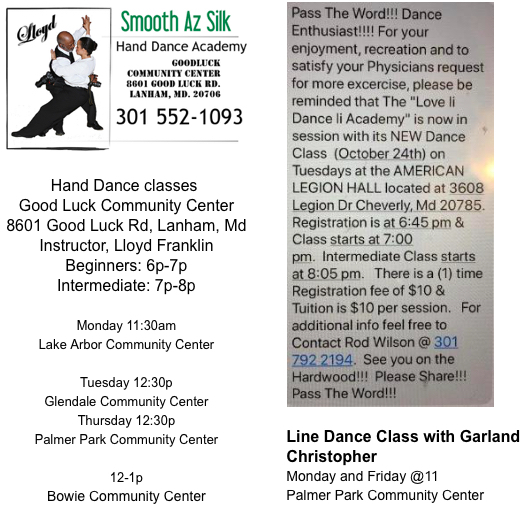table div table+table+table+table+table+table+table+table+table+table+table+table+table+table+table+table+table+table+table+table+table+table+table+table+table+table+table+table+table+table+table+table+table+table+table+table+table+table+table+table+table+table+table+table+table+table+table+table+table+table+table+table+table+table+table+table+table+table div table{width:100%;padding:0}table div table+table+table+table+table+table+table+table+table+table+table+table+table+table+table+table+table+table+table+table+table+table+table+table+table+table+table+table+table+table+table+table+table+table+table+table+table+table+table+table+table+table+table+table+table+table+table+table+table+table+table+table+table+table+table+table+table+table div table img{width:96.23%;padding:0;float:none}table div table+table+table+table+table+table+table+table+table+table+table+table+table+table+table+table+table+table+table+table+table+table+table+table+table+table+table+table+table+table+table+table+table+table+table+table+table+table+table+table+table+table+table+table+table+table+table+table+table+table+table+table+table+table+table+table+table+table div table td{width:100%;padding:0 1.88% 18px}/* styles *//* styles */ Denise's "Music2YourFeet" Line Dancing provides you with the opportunity to exercise socialize and have fun - all in one setting. Break the monotony of a long day at work, (evening classes); get mid-day relief to an otherwise stressful day (lunch time classes); Relax after a long work week (weekend classes); or one-on-one personal service (private classes, no matter the day, it will be music2yourfeet Submit your events. Donations not required but appreciated.
 table div table+table+table+table+table+table+table+table+table+table+table+table+table+table+table+table+table+table+table+table+table+table+table+table+table+table+table+table+table+table+table+table+table+table+table+table+table+table+table+table+table+table+table+table+table+table+table+table+table+table+table+table+table+table+table+table+table+table+table+table+table div table{width:100%;padding:0}table div table+table+table+table+table+table+table+table+table+table+table+table+table+table+table+table+table+table+table+table+table+table+table+table+table+table+table+table+table+table+table+table+table+table+table+table+table+table+table+table+table+table+table+table+table+table+table+table+table+table+table+table+table+table+table+table+table+table+table+table+table div table img{width:96.23%;padding:0;float:none}table div table+table+table+table+table+table+table+table+table+table+table+table+table+table+table+table+table+table+table+table+table+table+table+table+table+table+table+table+table+table+table+table+table+table+table+table+table+table+table+table+table+table+table+table+table+table+table+table+table+table+table+table+table+table+table+table+table+table+table+table+table div table td{width:100%;padding:0 1.88% 18px}/* styles */## Upcoming Events

 /* styles */ BTL Soul Line Dancers Present Summer Sensation 2019 July 13th, 2019 at the East County Rec Center, 3310 Gates Head Manor Way, Silver Spring Md, 20904, from 5:00pm to 10:00pm, \$10.00 G.O.L.D. Stress Free Line Dance Weekend, July 27, 2019, Glenarden Community Center 8615 McClain Ave, Glenarden Md 20706, from 6:00pm to 11:00pm, \$15.00 August 3rd, DC Dance & Wellness Presenters, Presented by: Decisive Media Network, Dance & Wellness Escape, Patuxent River 4H Center, 18405 Queen Anne Rd, Upper Marlboro 10:00am-8:00pm..Merging Dance & Wellness...Open to the public...flier will be in June newsletter,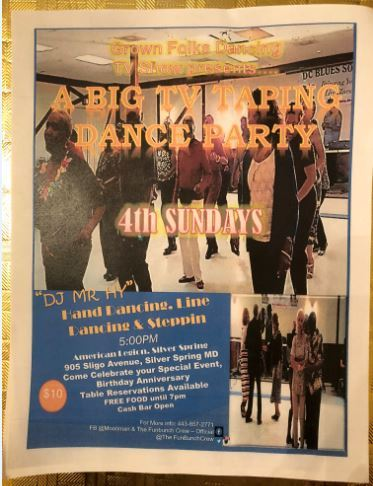table div table+table+table+table+table+table+table+table+table+table+table+table+table+table+table+table+table+table+table+table+table+table+table+table+table+table+table+table+table+table+table+table+table+table+table+table+table+table+table+table+table+table+table+table+table+table+table+table+table+table+table+table+table+table+table+table+table+table+table+table+table+table+table+table+table div table{width:100%;padding:0}table div table+table+table+table+table+table+table+table+table+table+table+table+table+table+table+table+table+table+table+table+table+table+table+table+table+table+table+table+table+table+table+table+table+table+table+table+table+table+table+table+table+table+table+table+table+table+table+table+table+table+table+table+table+table+table+table+table+table+table+table+table+table+table+table+table div table img{width:96.23%;padding:0;float:none}table div table+table+table+table+table+table+table+table+table+table+table+table+table+table+table+table+table+table+table+table+table+table+table+table+table+table+table+table+table+table+table+table+table+table+table+table+table+table+table+table+table+table+table+table+table+table+table+table+table+table+table+table+table+table+table+table+table+table+table+table+table+table+table+table+table div table td{width:100%;padding:0 1.88% 18px}/* styles */